# Deep Bar Double Cage Induction Motor

Generally in induction motor related operations, squirrel cage induction motors are widely used. The starting torque equation of an induction motor is given byWhere, R2 and X2 are the rotor resistance and inductive reactance at starting respectively, E2 is the rotor induced EMF and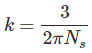Ns is the RPS speed of synchronous stator flux. Here in this equation the starting torque of induction motor Tsh is proportional to rotor resistance R2.

But the thing is that squirrel cage induction motor has very low starting torque due to its rotor resistance of very low value. So to provide a higher value of rotor resistance in squirrel cage induction motor double bar double cage rotor is used in induction motor.

The motive is to provide higher value of rotor resistance in such a manner that the rotor with its higher valued resistance provides higher torque and more efficiency.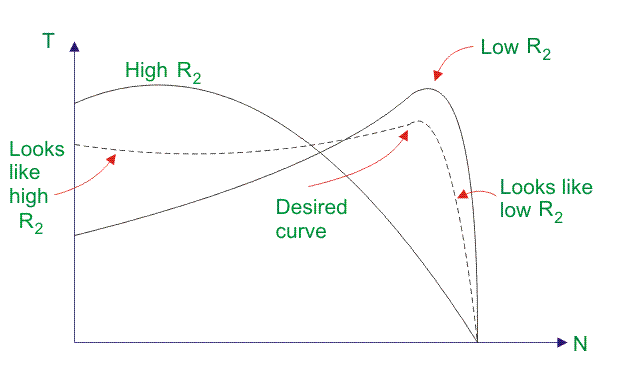## Why Starting Torque is Poor in Squirrel Cage Induction Motor?

The resistance cannot be varied in squirrel cage rotor as it is possible in slip ring induction motor. The fixed resistance of the rotor of the squirrel cage induction motor is very low. At the starting moment, the induced voltage in the rotor has same frequency as the frequency of the supply. Hence the starting inductive reactance gets higher value at stand still condition. The frequency of the rotor current gets same frequency as the supply frequency at standstill. Now the case is that the rotor induced current in spite of having higher value lags the induced voltage at a large angle. So this causes poor starting torque at the stand still condition. This torque is only 1.5 times of the full load torque though the induced current is 5 to 7 times of the full load current. Hence, this squirrel cage single bar single cage rotor is not being able to apply against high load. We should go for deep bar double cage induction motor to get higher starting torque.

### Construction of Deep Bar Double Cage Induction Motor

In deep bar double cage rotor bars are there in two layers.
Outer layer has the bars of small cross sections. This outer winding has relatively large resistance. The bars are shorted at the both ends. The flux linkage is thus very less. And hence inductance is very low. Resistance in outer squirrel cage is relatively high. Resistance to inductive reactance ration is high.

Inner layer has the bars of large cross section comparatively. The resistance is very less. But flux linkage is very high. The bars are thoroughly buried in iron. As flux linkage is high the inductance is also very high. The resistance to inductive reactance ration is poor.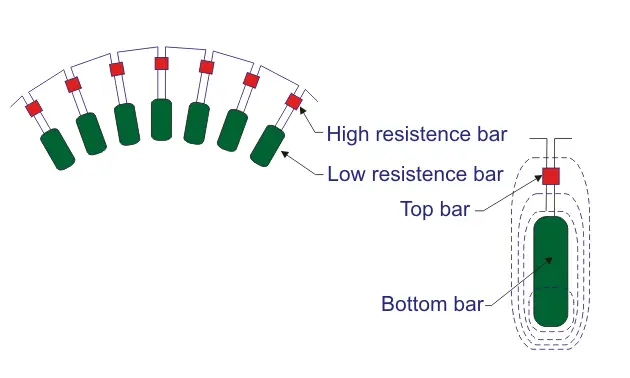### Operational Principle Construction of Deep Bar Double Cage Induction Motor

At the stand still condition the inner and outer side bars get induced with voltage and current with the same frequency of the supply. Now the case is that the inductive reactance (XL = 2πfL) is offered more in the deep bars or inner side bars due to skin effect of the alternating quantity i.e. voltage and current. Hence the current tries to flow through the outer side rotor bars.The outer side rotor offers more resistance but poor inductive reactance. The ultimate resistance is somewhat higher than the single bar rotor resistance. The higher valued rotor resistance results more torque to be developed at the starting. When the speed of the rotor of the deep bar double cage induction motor increases, the frequency of the induced EMF and current in the rotor gets gradually decreased. Hence the inductive reactance (XL) in the inner side bars or deep bars gets decreased and the current faces less inductive reactance and less resistance as a whole. Now no need for more torque because the rotor already has arrived to its full speed with running torque.

#### Speed Torque Characteristics of Deep Rotor IM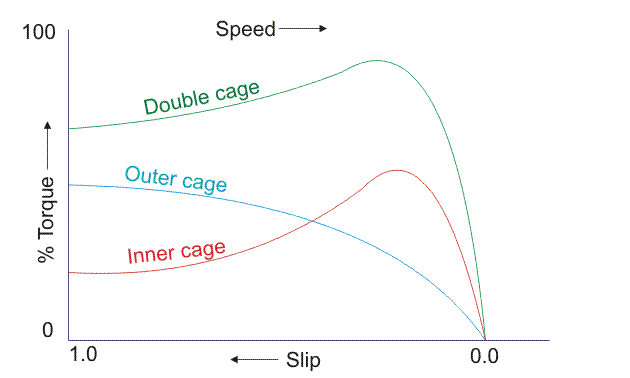Where, R2 and X2 are the rotor resistance and inductive reactance at starting respectively, E2 is the rotor induced EMF and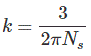Ns is the RPS speed of synchronous stator flux and S is the slip of the rotor speed. The above speed-torque graph shows that the higher valued resistance offers higher torque at the stand still condition and the max torque will be achieved at higher valued slip.

#### Comparison between Single Cage and Double Cage Motors

1. A double cage rotor has low starting current and high starting torque. Therefore, it is more suitable for direct on line starting.
2. Since effective rotor resistance of double cage motor is higher, there is larger rotor heating at the time of starting as compared to that of single cage rotor.
3. The high resistance of the outer cage increases the resistance of double cage motor. So full load copper losses are increased and efficiency is decreased.
4. The pull out torque of double cage motor is smaller than single cage motor.
5. The cost of double cage motor is about 20-30 % more than that of single cage motor og same rating.

Want To Learn Faster? 🎓
Get electrical articles delivered to your inbox every week.
No credit card required—it’s 100% free.